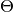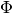3. Regularizers and Scores Usage¶

Detailed description of all parameters and methods of BigARTM Python API classes can be found in Python Interface. Description of regularizers can be found in Regularizers Description.

The library has a pre-defined set of the regularizers (you can create new ones, if it’s necessary, you can read about it in the corresponding notes in Creating New Regularizer). Now we’ll study to use them.

We assume that all the conditions from the head of the section 2. Base PLSA Model with Perplexity Score are executed. Let’s create the model and enable the perplexity score in it:

model = artm.ARTM(num_topics=20, dictionary=my_dictionary, cache_theta=False)
dictionary=my_dictionary))

I should note the the cache_theta flag: it’s allow you to save yourmatrix in the memory or not. If you have large collection, it can be impossible to store it’sin the memory, and in case of short collection it can be useful to look at it. Default value is False. In the cases, when you need to usematrix, but it is too big, you can use ARTM.transform() method (it will be discussed later).

Now let’s try to add other scores, because the perplexity is not the only one to be used.

Let’s add the scores of sparsity ofandmatrices and the information about the most probable tokens in each topic (top-tokens):

Scores have many useful parameters. For instance, they can be calculated on the subsets of topics. Let’s count separately the sparsity of the first ten topics in. But there’s a problem: topics are identifying with their names, and we didn’t specify them. If we used the topic_names parameter in the constructor (instead of num_topics one), we should have such a problem. But the solution is very easy: BigARTM had generated names and put them into the topic_names field, so you can use it:

Certainly, we could modify the previous score without creating new one, if the general model sparsity wasn’t interesting for us:

model.scores['sparsity_phi_score'].topic_names = model.topic_names[0: 9]

But let’s assume that we are also interested in it and keep everything as is. You should remember that all the parameters of metrics, model and regularizers (we will talk about them soon) can be set and reset by the direct change of the corresponding field, as it was demonstrated in the code above.

For example, let’s ask the top-tokens score to show us 12 most probable tokens in each topic:

model.num_tokens = 12

Well, we achieved the model covered with necessary scores, and can start the fitting process:

model.fit_offline(batch_vectorizer=batch_vectorizer, num_collection_passes=10)

We saw this code in the first section. But now we can see the values of new added scores:

print model.score_tracker['perplexity_score'].value      # .last_value
print model.score_tracker['sparsity_phi_score'].value    # .last_value
print model.score_tracker['sparsity_theta_score'].value  # .last_value

As we can see, all the scores didn’t change. But we forgot about the top-tokens. Here we need to act more accurately: the score stores the data on each moment ofupdate. Let’s assume that we need only the last data. So we need to use the last_tokens field. It is a Python dict, where key is a topic name, and value is a list of top-tokens of this topic.

Note

The scores are loading from the kernel on each call, so for such a big scores, as top-tokens (or topic kernel score), it’s strongly recommended to store the whole score in the local variable, and then deal with it. So, let’s look through all top-tokens in the loop:

saved_top_tokens = model.score_tracker['top_tokens_score'].last_tokens

for topic_name in model.topic_names:
print saved_top_tokens[topic_name]

Note

Here are several important facts about scores implementation:

• Each ARTM model has its three separate caches: one for cumulative scores, another for scores history (aka score_tracker) and a third one for caching theta matrix.
• The cache for scores only store cumulative scores (e.g. only scores that depend on theta matrix). Examples are: perplexity or ThetaMatrixSparsity.
• score_tracker contains the history for all scores (including non-cumulative scores). Note, that it cannot be used when fit_online method is called with async parameter set to True.
• Scores can be retrieved from the cache by ARTM.get_score(). This method can be also used to calculate a non-cumulative score for the current version of the Phi matrix.
• Score cache is reset at the beginning of fit_offline, fit_online and transform methods. As a result, get_score will always return the score calculated during the last call to fit_offline, fit_online or transform. For fit_online the score produced by get_score will be accumulated across all batches passed to fit_online.
• Score tracker is updated by fit_offline (adds one extra point) and fit_online (adds multiple points - as many as there were synchronizations). transform never adds points to score_tracker.
• Score tracker is never reset automatically. To reset the cache manually call ARTM.master.clear_score_array_cache.
• Theta matrix cache is updated by fit_offline, fit_online and transform methods. The cache contains one entry per batch. If batch with the same batch.id already exist in the cache the entry will be overwritten by a new theta matrix (for that batch).
• Theta matrix cache is reset at the beginning of transform method when transform is called with theta_matrix_type=Cache. This is the only case when theta cache is reset - all other transform calls, as well as calls to fit_offline / fit_online do not reset theta matrix cache.
• User may reset theta matrix cache by calling ARTM.remove_theta().
• User may also reset score cache by calling ARTM.master.clear_score_cache.

The code for computation of held-out perplexity can be found in 5. Phi and Theta Extraction. Transform Method.

Probably the topics are not very good. For the aim of increasing the quality of the topics you can use the regularizers. The code for dealing with the regularizers is very similar with the one for scores. Let’s add three regularizers into our model: sparsing ofmatrix, sparsing ofmatrix and topics decorrelation. The last one is need to make topics more different.

Maybe you have a question about the name of the SmoothSparsePhi\Theta regularizer. Yes, it can both smooth and sparse topics. It’s action depends on the value of corresponding coefficient of the regularization :math:tau (we assume, that you know, what is it). tau > 0 leads to smoothing, tau < 0 to sparsing. By default all the regularizers has tau = 1, which is usually not what you want. Choosing good tau is a heuristic, sometimes you need to process dozens of the experiments to pick up good values. It is the experimental work, and we won’t discuss it here. Let’s look at technical details instead:

model.regularizers['sparse_phi_regularizer'].tau = -1.0
model.regularizers['sparse_theta_regularizer'].tau = -0.5
model.regularizers['decorrelator_phi_regularizer'].tau = 1e+5

We set standard values, but in bad case they can be useless or even harmful for the model.

We draw your attention again to the fact, that setting and changing the values of the regularizer parameters is fully similar to the scores.

Let’s start the learning process:

model.fit_offline(batch_vectorizer=batch_vectorizer, num_collection_passes=10)

Further you can look at metrics, change tau coefficients of the regularizers and etc. As for scores, you can ask the regularizer to deal only with given topics, using topic_names parameter.

Let’s return to the dictionaries. But here’s one discussion firstly. Let’s look at the principle of work of the SmoothSparsePhi regularizer. It simply adds to all counters the same value tau. Such a strategy can be unsuitable for us. The probable case: a need for sparsing one part of words, smoothing another one and ignoring the rest tokens. For example, let’s sparse the tokens about magic, smooth tokens about cats and ignore all other ones.

In this situation we need dictionaries.

Let’s remember about the value field, that corresponds each unique token. And also the fact, that SmoothSparsePhi regularizer has the dictionary field. If you set this field, the regularizer will add to counters tau * value for this token, instead of tau. In such way we can set the tau to 1, for instance, set the value variable in dictionary for tokens about magic equal to -1.0, for tokens about cats equal to 1.0, and 0.0 for other tokens. And we’ll get what we need.

The last problem is how to change these value variables. It was discussed in the 1. Loading Data: BatchVectorizer and Dictionary: let’s remember about the methods Dictionary.save_text() and Dictionary.load_text().

You need to proceed next steps:

• save the dictionary in the textual format;
• open it, each line corresponds to one unique token, the line contains 5 values: token - modality - value - token_tf - token_df;
• don’t pay attention to anything except the token and the value; find all tokens you are interested in and change their values parameters;
• load the dictionary back into the library.

Your file can have such a view after editing (conceptually):

cat      smth      1.0      smth      smth
shower   smth      0.0      smth      smth
magic    smth     -1.0      smth      smth
kitten   smth      1.0      smth      smth
merlin   smth     -1.0      smth      smth
moscow   smth      0.0      smth      smth

All the code you need to process discussed operation was showed above. Here is an example of creation of the regularizer with dicitonary: